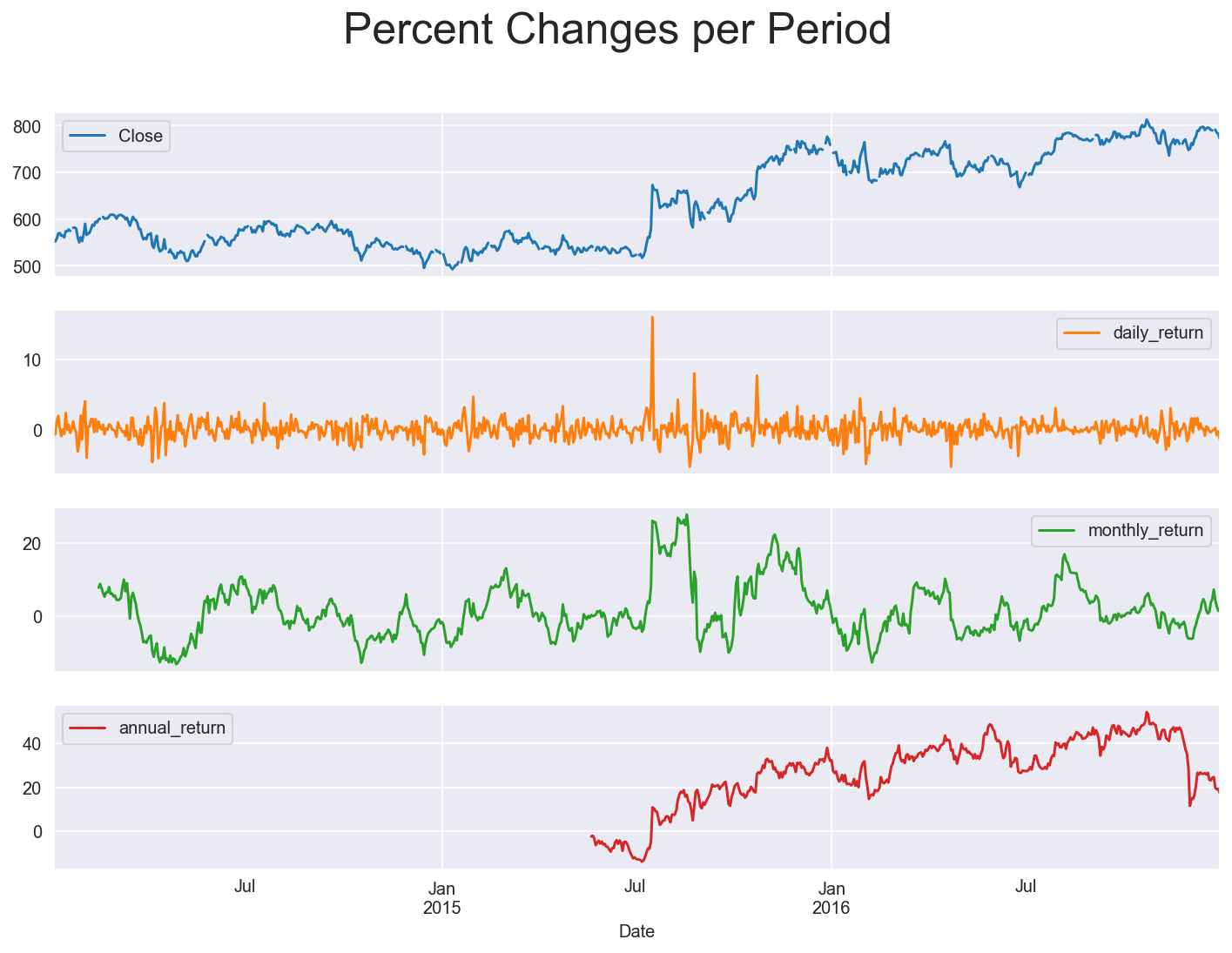Back to Templates

# Calculate Percent Changes, Lags and Shifts on Time Series

This template helps you calculate and visualize the percent changes over different time periods. Furhtermore it helps you shift or lag your time series data forward or backward.

Typical applications for these time series manipulations range from monitoring sensor data, tracking financial assets and analyzing and predicting shopping behavior to studying cyclical events like planetary movement.

```.css-y3yaiy{border:0;border-radius:4px;color:rgb(5, 25, 45);font-family:monospace;font-family:JetBrainsMonoNL;font-size:14px;font-variant-ligatures:none;letter-spacing:0;line-height:1.5;margin:0;padding:0;}```# Load packages
import pandas as pd
import matplotlib.pyplot as plt
import plotly.graph_objects as go
import seaborn as sns
sns.set_style('darkgrid')
%config InlineBackend.figure_format = 'retina'
plt.rcParams['figure.figsize'] = (12,8)
plt.rcParams["legend.loc"] = 'upper left'``````
``````# Upload your data as CSV and load as data frame
parse_dates = ['Date'],       # Specify date column(s)
index_col = 'Date')           # Specify which column to take as index
Close
Date
2014-01-02556.00
2014-01-03551.95
2014-01-04NaN
2014-01-05NaN
2014-01-06558.10
``````# Set data frequency to business daily
df = df.asfreq('B')                               # Change datetime frequency

# Create 'lagged' and 'shifted'
df['lag_90'] = df['Close'].shift(-90)             # Create a lag (backward)
df['shift_90'] = df['Close'].shift(90)            # Create a shift (forward)

# Plot the google price series
df.plot(subplots=True, legend=True)
plt.suptitle("Stock price with lag and shift",    # Set plot title
size=25)
plt.show()

# Drop newly created rows
df.drop(['lag_90', 'shift_90'], axis=1, inplace=True)````````````# Create daily_return
df['daily_return'] = df['Close'].pct_change(1).mul(100)

# Create monthly_return
df['monthly_return'] = df['Close'].pct_change(30).mul(100)

# Create annual_return
df['annual_return'] = df['Close'].pct_change(360).mul(100)

# Plot percent changes per period
df.plot(subplots=True)
plt.suptitle('Percent Changes per Period', size=25)
plt.show()``````### Calculate Percent Changes, Lags and Shifts on Time Series

Calculate shifts and lags and visualize the percent changes over different time periods.

Use Template